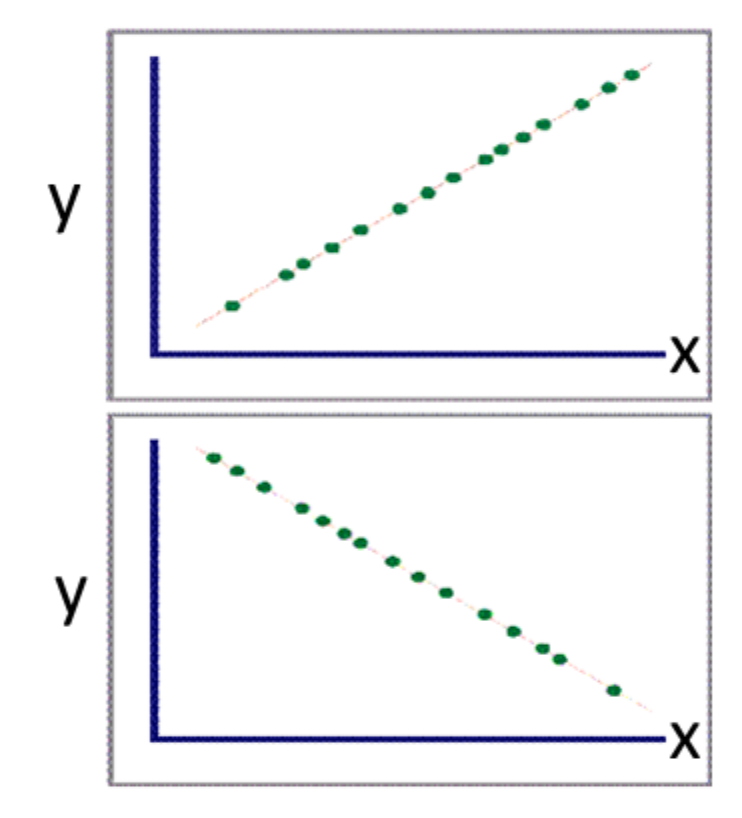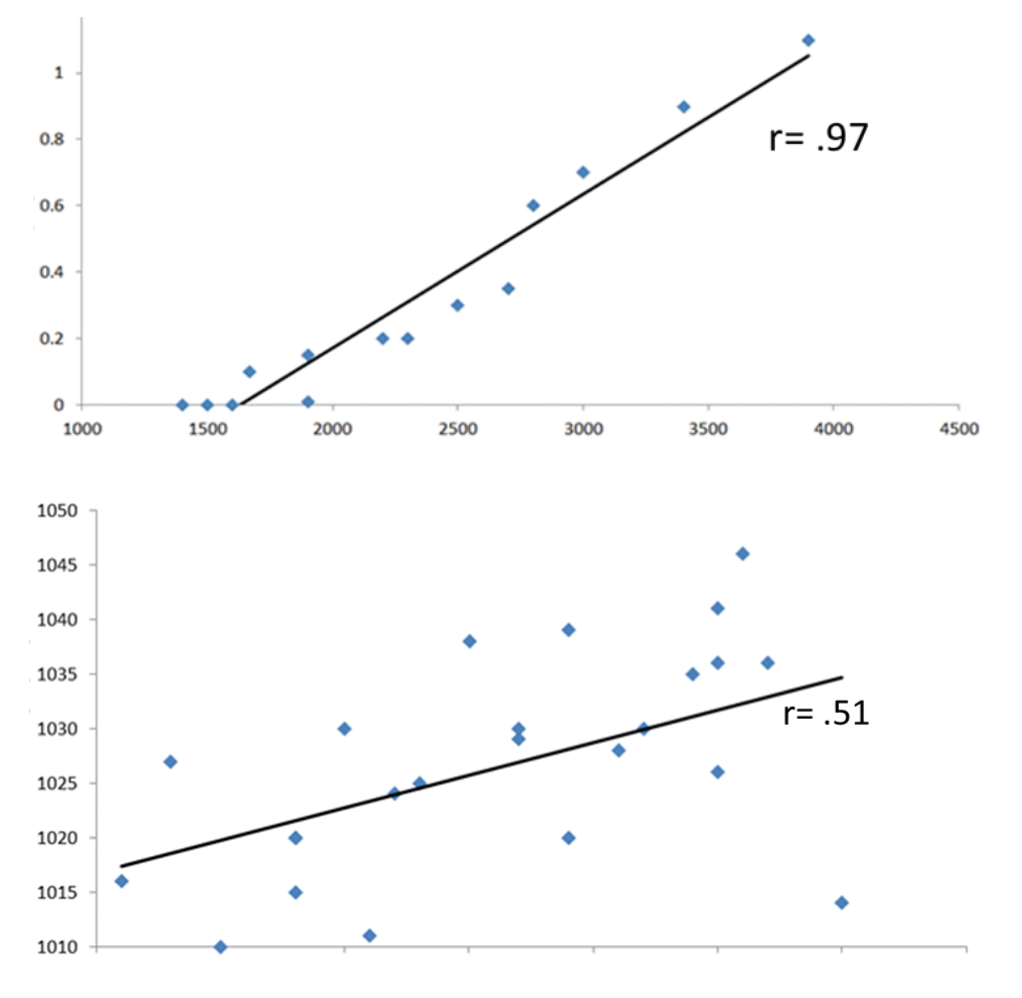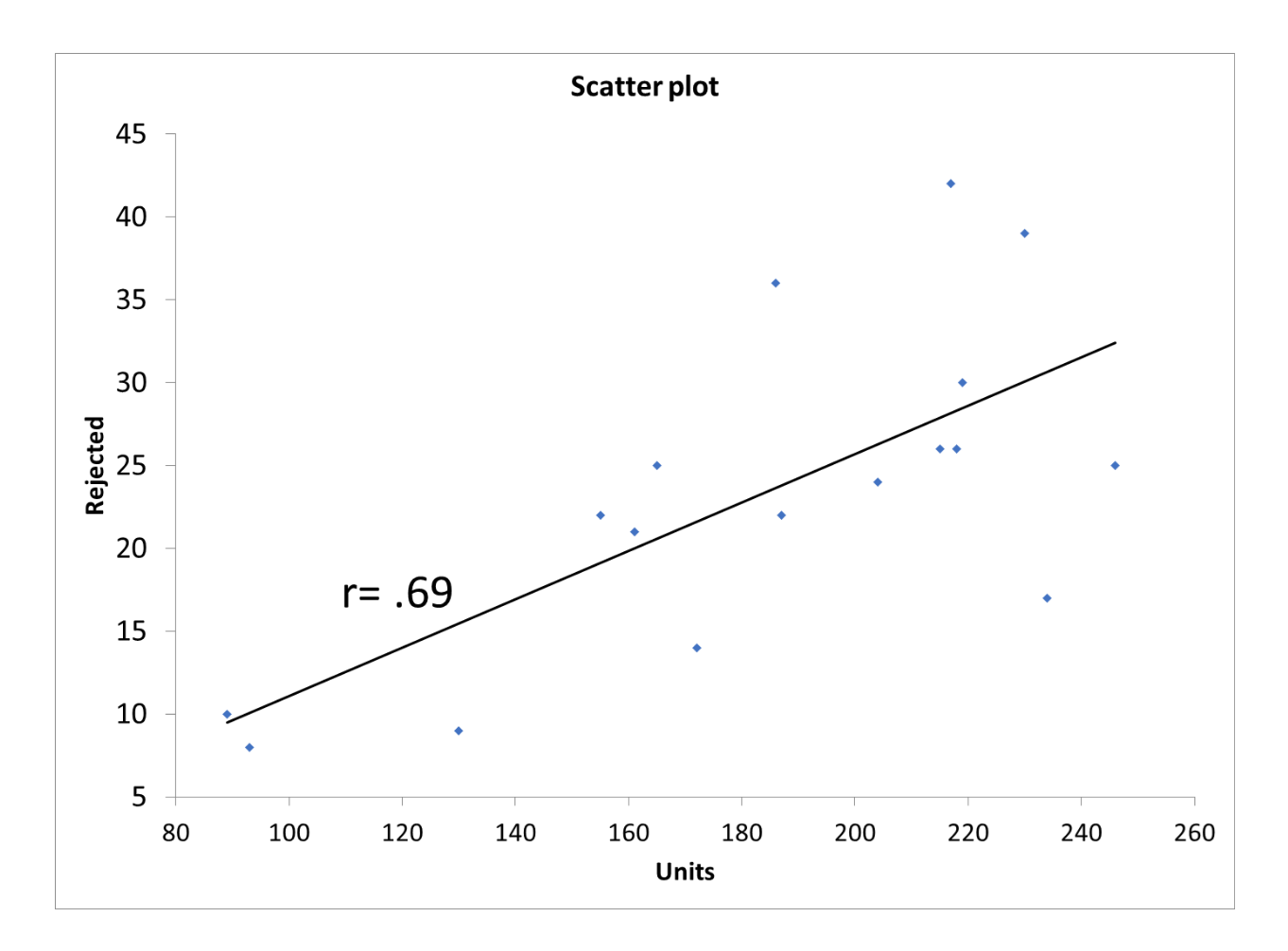The term linear relationship seems to infer a relationship that would follow a line, hence the term linear. You can also have a curvilinear relationship that would follow a curve. Let’s explore the concept of a linear relationship.

## Overview: What is a linear relationship?

The concept of a linear relationship between two variables usually comes up in the context of simple linear regression. In simple linear regression, you are attempting to establish whether the relationship between your X and Y variables is linear. This would mean as your X variable goes up, your Y variable would go up or down in sync with your X variable. A perfect linear relationship would have your Y go up or down one unit as your X went up one unit.

If your X goes up one unit and your Y goes up one unit we would say your linear relationship would have a positive correlation. If your X went up one unit and your Y went down one unit, you would have a negative correlation. The two scatter plots below show a perfect positive and negative linear relationship or correlation.It is very rare your data will present as a perfect linear relationship or correlation. A common measure for determining the degree of linear relationship is the Pearson correlation coefficient. Values for the Pearson correlation designated as r, will range between -1.0 (perfect negative linear) and +1.0 (perfect positive linear) with 0 meaning no linear relationship or correlation. The closer to 1 on either side indicates how strong the linear relationship is. Below are two scatter plots showing a strong and weak linear relationship based on the graphical relationship to a straight line and the value of the Pearson correlation.## An industry example of a linear relationship

The plant manager for a glass company believed there was a linear relationship between the number of units produced and the number of rejected units. He did a quick scatter plot and while visually it doesn’t appear to be a strong linear relationship, the r value of 0.69 showed it to be relatively strong. The scatter plot below shows the output from his statistical software.### What does a strong linear relationship look like on a graph?

All the points will fall on or near the calculated regression line.

### Is there a way to measure the statistical strength of a linear relationship?

Yes. The Pearson correlation is a common statistical calculation measuring the strength of the linear relationship. Its values range between -1 and +1. A value of zero means there is no linear relationship. The closer the value is to 1, either positive or negative, the stronger the linear relationship.

### What is the Coefficient of Determination?

The Coefficient of Determination, written as r-square, is the square of the Pearson Correlation Coefficient. It defines the percent variation in your Y that is explained by your X.